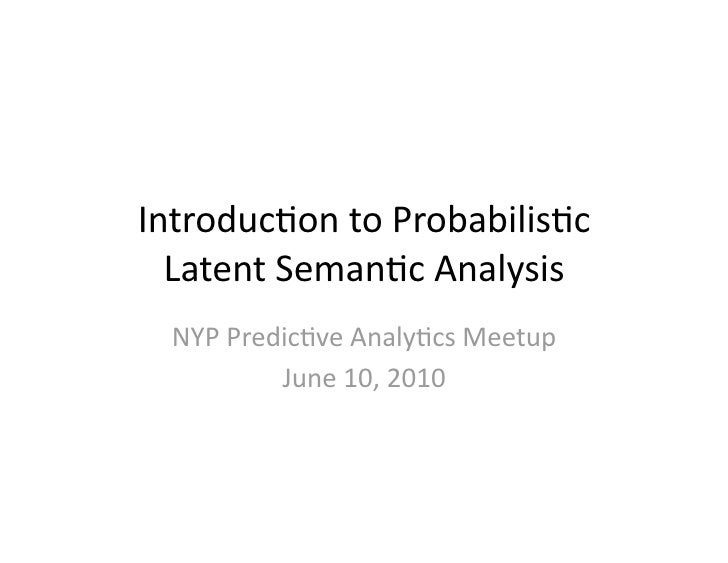Successfully reported this slideshow.Upcoming SlideShare
×

# Introduction to Probabilistic Latent Semantic Analysis

11,177 views

Published on

• Full Name
Comment goes here.

Are you sure you want to Yes No• thx for gentle explanation

Are you sure you want to  Yes  No

as presented to NYC Predictive Analytics Meetup on June 10, 2010 by John Stewart, VP of Research and Analytics at Wireless Generation and a doctoral student at the CUNY Graduate Center focusing on applications of computational linguistics. John holds a BA from Yale.

Are you sure you want to  Yes  No

### Introduction to Probabilistic Latent Semantic Analysis

1. 1. Introduc)on to Probabilis)c  Latent Seman)c Analysis  NYP Predic)ve Analy)cs Meetup  June 10, 2010
2. 2. PLSA  •  A type of latent variable model with observed  count data and nominal latent variable(s).  •  Despite the adjec)ve ‘seman)c’ in the acronym,  the method is not inherently about meaning.  –  Not any more than, say, its cousin Latent Class  Analysis  •  Rather, the name must be read as P + LS(A|I),  marking the genealogy of PLSA as a probabilis)c  re‐cast of Latent Seman)c Analysis/Indexing.
3. 3. LSA  •  Factoriza)on of data matrix into orthogonal  matrices to form bases of (seman)c) vector  space:  •  Reduc)on of original matrix to lower‐rank:  •  LSA for text complexity: cosine similarity between  paragraphs.
4. 4. Problems with LSA  •  Non‐probabilis)c  •  Fails to handle polysemy.    –  Polysemy called “noise” in LSA literature.  •  Shown (by Hofmann) to underperform  compared to PLSA on IR task
5. 5. Probabili)es Why?  •  Probabilis)c systems allow for the evalua)on of  proposi)ons under condi)ons of uncertainty.   Probabilis)c seman)cs.  •  Probabilis)c systems provide a uniform mechanism for  integra)ng and reasoning over heterogeneous  informa)on.  –  In PLSA seman)c dimensions are represented by unigram  language models, more transparent than eigenvectors.  –  The latent variable structure allows for subtopics  (hierarchical PLSA)  •  “If the weather is sunny tomorrow and I’m not )red we  will go to the beach”  –  p(beach) = p(sunny & ~)red) = p(sunny)(1‐p()red))
6. 6. A Genera)ve Model?  •  Let X be a random vector with components {X1,  X2, … , Xn} random variables.  •  Each realiza)on of X is assigned to a class, one of  a random variable Y.  •  A genera(ve model tells a story about how the  Xs came about: “once upon a )me, a Y was  selected, then Xs were created out of that Y”.  •  A discrimina(ve model strives to iden)fy, as  unambiguously as possible, the Y value for some  given X
7. 7. A Genera)ve Model?  •  A discrimina)ve model es)mates P(Y|X)  directly.  •  A genera)ve model es)mates P(X|Y) and P(Y)  –  The predic)ve direc)on is then computed via  Bayesian inversion:   where P(X) is obtained by condi)oning on Y:
8. 8. A Genera)ve Model?  •  A classic genera)ve/discrimina)ve pair: Naïve  Bayes vs Logis)c Regression.  •  Naïve Bayes assumes that the Xis are  condi)onally independent given Y, so it es)mates  P(Xi | Y).  •  Logis)c regression makes other assump)ons, e.g.  linearity of the independent variables with logit  of dependent, independence of errors, but  handles correlated predictors (up to perfect  collinearity).
9. 9. A Genera)ve Model?  •  Genera)ve models have richer probabilis)c  seman)cs.    –  Func)ons run both way.  –  Assign distribu)ons to the “independent” variables,  even previously unseen realiza)ons.  •  Ng and Jordan (2002) show that logis)c  regression has higher asympto)c accuracy, but  converges more slowly, sugges)ng a trade‐oﬀ  between accuracy and variance.  •  Overall trade‐oﬀ between accuracy and  usefulness.
10. 10. A Genera)ve Model?  •  Start with document  •  Start with topic  D  P(D)  P(D|Z)  D  Z  Z  P(Z|D)  P(Z)  W  P(W|Z)  W  P(W|Z)
11. 11. A Genera)ve Model?  •  The observed data are cells of document‐term matrix  –  We generate (doc, word) pairs.  –  Random variables D, W and Z as sources of objects  •  Either:  –  Draw a document, draw a topic from the document, draw  a word from the topic.  –  Draw a topic, draw a document from the topic, draw a  word from the topic.  •  The two models are sta)s)cally equivalent  –  Will generate iden)cal likelihoods when ﬁt  –  Proof by Bayesian inversion  •  In any case D and W are condi)onally independent  given Z.
12. 12. A Genera)ve Model?
13. 13. A Genera)ve Model?  •  But what is a Document here?  –  Just a label!  There are no anributes associated with  documents.    –  P(D|Z) relates topics to labels  •  A previously unseen document is just a new label  •  Therefore PLSA isn’t genera)ve in an interes)ng  way, as it cannot handle previously unseen inputs  in a genera)ve manner.  –  Though the P(Z) distribu)on may s)ll be of interest.
14. 14. Es)ma)ng the Parameters  •  Θ = {P(Z); P(D|Z); P(W|Z)}  •  All distribu)ons refer to latent variable Z, so  cannot be es)mated directly from the data.  •  How do we know when we have the right  parameters?  –  When we have the θ that most closely generates  the data, i.e. the document‐term matrix
15. 15.  Es)ma)ng the Parameters  •  The joint P(D,W) generates the observed  document‐term matrix.  •  The parameter vector θ yields the joint P(D,W)  •  We want θ that maximizes the probability of  the observed data.
16. 16. Es)ma)ng the Parameters  •  For the mul)nomial distribu)on,  •  Let X be the MxN document‐term matrix.
17. 17. Es)ma)ng the Parameters  •  Imagine we knew the X’ = MxNxK complete  data matrix, where the counts for topics were  overt.  Then,  New and interes)ng:  The usual parameters θ  unseen counts must sum  to 1 for given d,w
18. 18. Es)ma)ng the Parameters  •  We can factorize the counts in terms of the  observed counts and a hidden distribu)on:  •  Let’s give the hidden distribu)on its name:  P(Z|D,W), the posterior distribu)on of Z w.r.t.  D,W
19. 19. Es)ma)ng the Parameters  •  P(Z|D,W) can be obtained from the  parameters via Bayes and our core model  assump)on of condi)onal independence:
20. 20. Es)ma)ng the Parameters  •  Nobody said the genera)on of P(Z|D,W) must  be based on the same parameter vector as the  one we’re looking for!  •  Say we obtain P(Z|D,W) based on randomly  generated parameters θn :  •  We get a func)on of the parameters:
21. 21. Es)ma)ng the Parameters  •  The resul)ng func)on, Q(θ), is the condi)onal  expecta)on of the complete data likelihood with  respect to the distribu)on P(Z|D,W).   •  It turns out that if we ﬁnd the parameters that  maximize Q we get a bener es)mate of the  parameters!  •  Expressions for the parameters can be had by  sesng the par)al deriva)ves with respect to the  parameters to zero and solving, using Laplace  transforms.
22. 22. Es)ma)ng the Parameters  •  E‐step (misnamed)  •  M‐step
23. 23. Es)ma)ng the Parameters  •  Concretely, we generate (randomly)    θ1 = {Pθ1(Z); Pθ1(D|Z); Pθ1(W|Z)} .   •  Compute the posterior Pθ1(Z|W,D).  •  Compute new parameters θ2 .   •  Repeat un)l “convergence”, say un)l the log  likelihood stops changing a lot, or un)l  boredom, or some N itera)ons.  •  For stability, average over mul)ple starts,  varying numbers of topics.
24. 24. Folding In  •  When a new document comes along, we want to  es)mate the posterior of the topics for the  document.  –  What is it about?  I.e. what is the distribu)on over  topics of the new document?  •  Perform a “linle EM”:   –  E‐step: compute P(Z|W, Dnew)  –  M‐step: compute P(Z|Dnew) keeping all other  parameters unchanged.  –  Converges very fast, ﬁve itera)ons?  –  Overtly discrimina)ve!  The true colors of the method  emerge.
25. 25. Problems with PLSA  •  Easily huge number of parameters  –  Leads to unstable es)ma)on (local maxima).  –  Computa)onally intractable because of huge  matrices  –  Modeling the documents directly can be problem  •  What if the collec)on has millions of documents?  •  Not properly genera)ve (is this a problem?)
26. 26. Examples of Applica)ons  •  Informa)on Retrieval: compare topic  distribu)ons for documents and queries using  a similarity measure like rela)ve entropy.  •  Collabora)ve Filtering (Hoﬀman, 2002) using  Gaussian PLSA.  •  Topic segmenta)on in texts, by looking for  spikes in the distances between topic  distribu)ons for neighbouring text blocks.